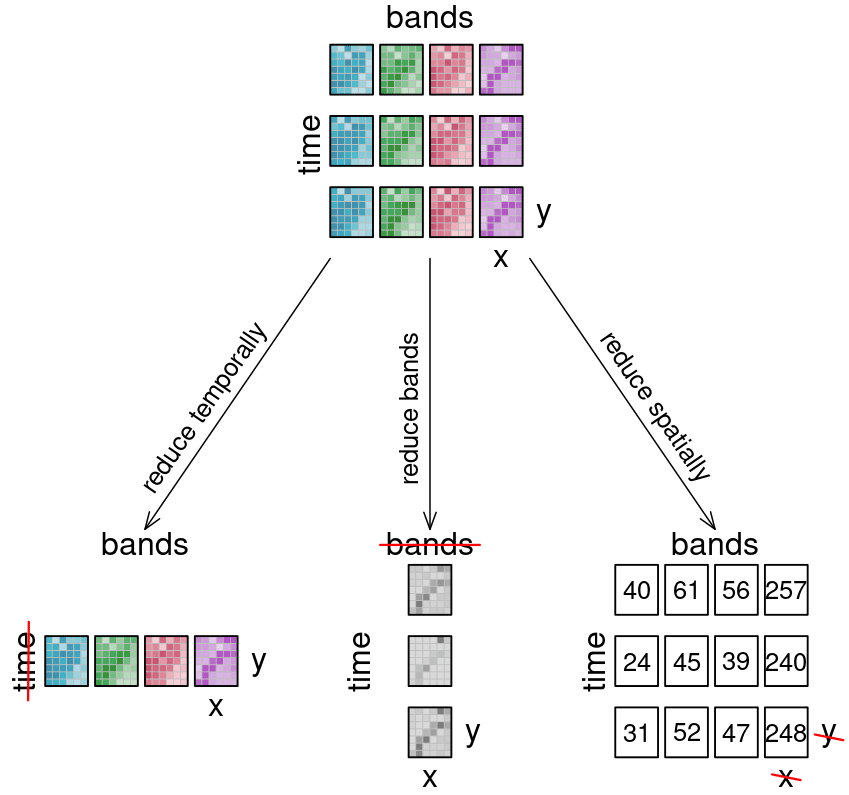Lesson 6, Topic 4
In Progress

# Reduce

##### EO College
Lesson Progress
0% Complete

The reduce_dimension process collapses a whole dimension of the datacube. It does so by using some sort of reducer, which is a function that calculates a single result from an amount of values, as e.g. mean()min() and max() are. For example we can reduce the time dimension (t) of a timeseries by calculating the mean value of all timesteps for each pixel. We are left with a cube that has no time dimension, because all values of that dimension are compressed into a single mean value. The same goes for e.g. the spatial dimensions: If we calculate the mean along the x and y dimensions, we are left without any spatial dimensions, but a mean value for each instance that previously was a raster is returned. In the image below, the dimensions that are reduced are crossed out in the result.

::: tip Simplified reduce([🥬, 🥒, 🍅, 🧅], prepare) => 🥗 :::

Think of it as a waste press that does math instead of using brute force. Given a representation of our example datacube, let’s see how it is affected.Datacube reduce: Three arrows depict what happens to the 12 example tiles, if they are reduced: Reducing timesteps leads to four tiles (one for each band), and the time dimension is deleted. Reducing bands lead to one tile per timestep, and the bands dimension is deleted. Reducing spatially leads to the original 4 by 3 bands by time layout, but the result has no spatial dimension and thus, the tiles have been turned into single values, per tile.

Learn how to use Reduce operators with this interactive exercise: